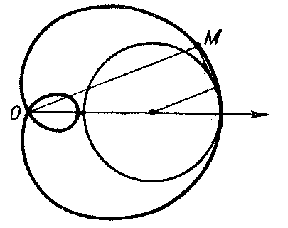# Pedal curve

of a curve $l$ with respect to a point $O$

The set of bases to the perpendiculars dropped from the point $O$ to the tangents to the curve $l$. For example, the Pascal limaçon is the pedal of a circle with respect to the point $O$( see Fig.). The pedal (curve) of a plane curve $x = x( t) , y = y( t)$ relative to the coordinate origin is

$$X = x - x ^ \prime \frac{xx ^ \prime + yy ^ \prime }{x ^ \prime 2 + y ^ \prime 2 } ,\ \ Y = y - y ^ \prime \frac{xx ^ \prime + yy ^ \prime }{x ^ \prime 2 + y ^ \prime 2 } .$$Figure: p071950a

The equation for the pedal of a curve $x= x( t), y= y( t), z= z( t)$ in space relative to the origin is

$$X = x - x ^ \prime \frac{xx ^ \prime + yy ^ \prime + zz ^ \prime }{x ^ \prime 2 + y ^ \prime 2 + z ^ \prime 2 } ,\ \ Y = y - y ^ \prime \frac{xx ^ \prime + yy ^ \prime + zz ^ \prime }{x ^ \prime 2 + y ^ \prime 2 + z ^ \prime 2 } ,$$

$$Z = z - z ^ \prime \frac{xx ^ \prime + yy ^ \prime + zz ^ \prime }{x ^ \prime 2 + y ^ \prime 2 + z ^ \prime 2 } .$$

The antipedal of a curve $l$ with respect to a point $O$ is the name given to the curve with as pedal, with respect to the point $O$, the curve $l$.

The pedal of a surface with respect to a point $O$ is the set of bases to the perpendiculars dropped from the point $O$ to the tangent planes to the surface. The equation for the pedal of a surface $F( x, y, z) = 0$ with respect to the coordinate origin is

$$X = F _ {x} \Phi ,\ \ Y = F _ {y} \Phi ,\ \ Z = F _ {z} \Phi ,$$

where

$$\Phi = \frac{xF _ {x} + yF _ {y} + zF _ {z} }{F _ {x} ^ { 2 } + F _ {y} ^ { 2 } + F _ {z} ^ { 2 } } .$$